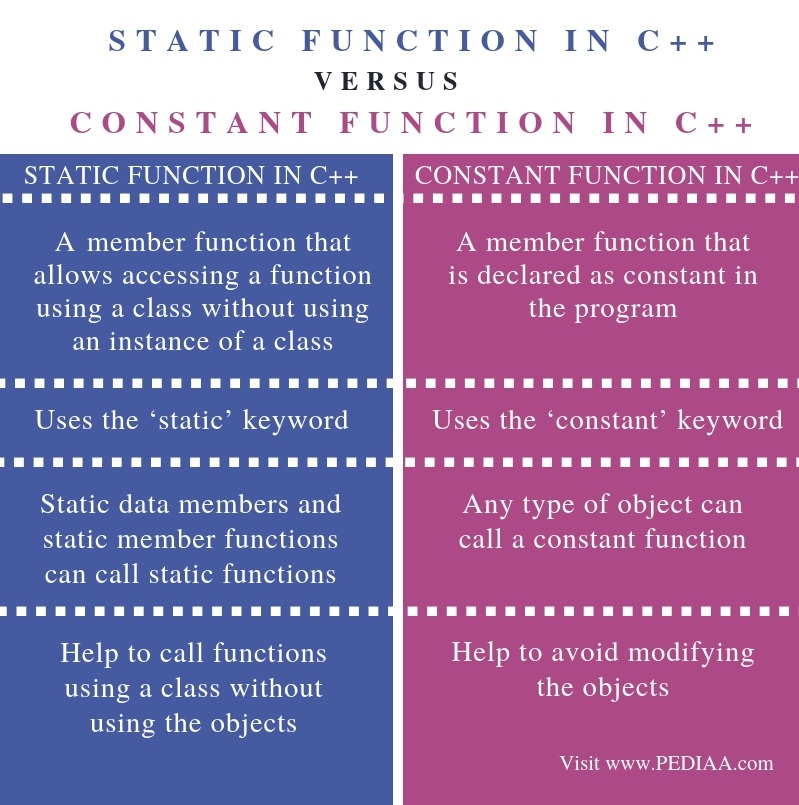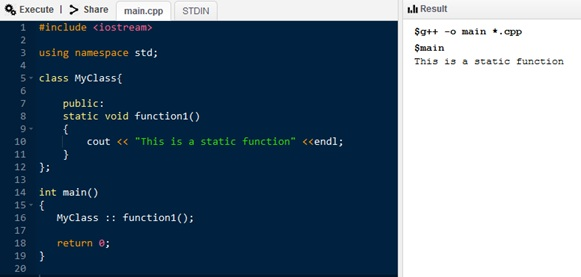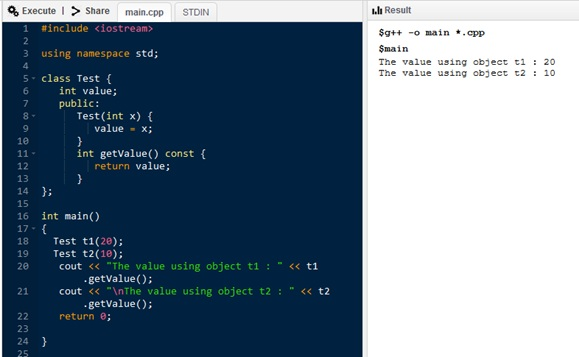# What is the Difference Between Static and Constant Function in C++

The main difference between Static and Constant function in C++ is that the Static function allows calling functions using the class, without using the object, while the Constant function does not allow modifying objects.

C++ is a programing language developed by Bjarne Stroustrup in 1979. C++ is similar to C but has more features than C. Therefore, it is called a subset of C language. The main advantage of C++ is that it supports Object Oriented Programming (OOP). The programmers can easily model the real world scenarios in computing using OOP. In OOP, everything is an object, and these objects communicate with each other. However, there should be a class to create objects. A class is a blueprint to create objects. Each class contains properties and behaviors, and properties describe the attributes or data while the methods or functions describe the behaviors. The attributes are member variables, and the functions are member functions. Static and constant functions are two types of functions.

### Key Areas Covered

1. What is Static Function in C++
– Definition, Functionality
2. What is Constant Function in C++
– Definition, Functionality
3. What is the Difference Between Static and Constant Function in C++
– Comparison of Key Differences

### Key Terms

Static Function, Constant Function, C++## What is Static Function in C++

A function defined with the static keyword is a static function. Usually, functions work with objects. Programmers use objects to call these functions. However, it is different in static functions. They work in class level, not for a particular object of a class. The programmer can call a static function using the class name and the scope resolution:: operator. A static function cannot access the usual data members and functions. It can only call static data members and static member functions.Figure 1: Static Function in C++

In the above program, there is a class called MyClass. It has a static function called fucntion1. In the main method, the programmer can call this static function by using the class name and the scope resolution as in line 16. When executing the program, it executes the static function, and prints the statement inside that function on the screen.

## What is Constant Function in C++

A function with the ‘const’ keyword is a constant function. The constant function does not allow modifying objects and related data members.Figure 2: Constant Function in C++

In the above figure, Test is a class. It has a data member called value. The Test constructor obtains x and sets that x to the data member value. Furthermore, there is a constant function, which returns the value. In the main program, t1 and t2 are objects of type Test. The getValue statements print the corresponding values of t1 and t2. As it a constant function, it does not allow performing any modification on the object.

## Difference Between Static and Constant Function in C++

### Definition

A static function is a member function that allows accessing a function using a class without using an instance of a class. A constant function is a member function that is declared as constant in the program. Thus, this is the main difference between Static and Constant Function in C++.

### Keywords

A static function uses the ‘static’ keyword whereas a constant function uses the ‘constant’ keyword.

### Data Members and Functions

Moreover, another difference between Static and Constant function in C++ is that the static data members and static member functions can call static functions whereas any type of object can call a constant function.

### Usage

Static functions help to call functions using a class without using the objects while Constant functions help to avoid modifying the objects. Hence, this is also a difference between Static and Constant function in C++.

### Conclusion

There are data (data members) and functions (member functions) in a class. Two types of member functions are static and constant functions. The main difference between Static and Constant function in C++ is that the Static function allows calling functions using a class, without using the object, while the Constant function does not allow modifying objects.

##### Reference:

1. “Types of Class Member Functions in C .” Types of Network Topology in Computer Networks | Studytonight, Available here.